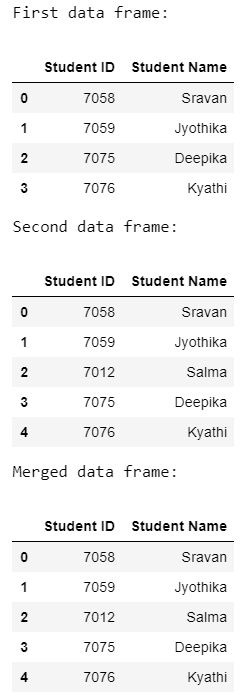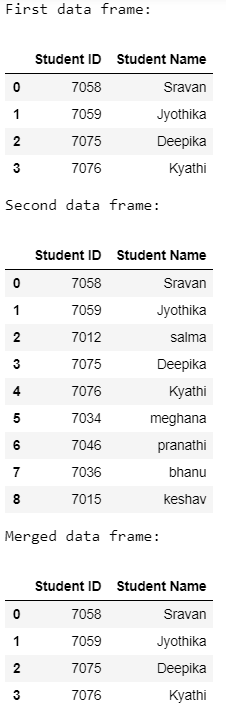GeeksforGeeks App
Open AppBrowser
Continue

# How to Merge DataFrames of different length in Pandas ?

In this article, we will discuss how to merge the two dataframes with different lengths in Pandas. It can be done using the merge() method.

Syntax:

DataFrame.merge(parameters)

Below are some examples that depict how to merge data frames of different lengths using the above method:

Example 1:

Below is a program to merge two student data frames of different lengths.

## Python3

 `# importing pandas module`` ` `import` `pandas as pd`` ` `# create a list that contains ``# student id of subject 1``list1 ``=` `[``7058``, ``7059``, ``7075``, ``7076``]`` ` `# create a list that contains``# student id of subject 2``list2 ``=` `[``7058``, ``7059``, ``7012``, ``7075``, ``7076``]`` ` `# create a list that contains ``# student names of subject 1``list11 ``=` `[``"Sravan"``, ``"Jyothika"``, ``"Deepika"``,``          ``"Kyathi"``]`` ` `# create a list that contains ``# student names of subject 2``list22 ``=` `[``"Sravan"``, ``"Jyothika"``, ``"Salma"``, ``          ``"Deepika"``, ``"Kyathi"``]`` ` ` ` `# pass list1 and list11 to the``# dataframe1``dataframe1 ``=` `pd.DataFrame(``  ``{``"Student ID"``: list1, ``"Student Name"``: list11})``print``(``'First data frame:'``)``display(dataframe1)`` ` `# pass list2 and list22 to the``# dataframe1``dataframe2 ``=` `pd.DataFrame(``  ``{``"Student ID"``: list2, ``"Student Name"``: list22})``print``(``'Second data frame:'``)``display(dataframe2)`` ` `# apply merge function to merge the``# two dataframes``mergedf ``=` `dataframe2.merge(dataframe1, how``=``'left'``)``print``(``'Merged data frame:'``)``display(mergedf)`

Output:Example 2:

Here is another program to merge one data frame of length 4 and another dataframe of length 9.

## Python3

 `# importing pandas module``import` `pandas as pd`` ` `# create a list that contains``# student id of subject 1``list1 ``=` `[``7058``, ``7059``, ``7075``, ``7076``]`` ` `# create a list that contains``# student id of subject 2``list2 ``=` `[``7058``, ``7059``, ``7012``, ``7075``, ``7076``,``         ``7034``, ``7046``, ``7036``, ``7015``]`` ` `# create a list that contains``# student names of subject 1``list11 ``=` `[``"Sravan"``, ``"Jyothika"``, ``"Deepika"``,``          ``"Kyathi"``]`` ` `# create a list that contains``# student names of subject 2``list22 ``=` `[``"Sravan"``, ``"Jyothika"``, ``"salma"``, ``          ``"Deepika"``, ``"Kyathi"``, ``"meghana"``,``          ``"pranathi"``, ``"bhanu"``, ``"keshav"``]`` ` ` ` `# pass list1 and list11 to the``# dataframe1``dataframe1 ``=` `pd.DataFrame(``  ``{``"Student ID"``: list1, ``"Student Name"``: list11})``print``(``'First data frame:'``)``display(dataframe1)`` ` `# pass list2 and list22 to the ``# dataframe1``dataframe2 ``=` `pd.DataFrame(``  ``{``"Student ID"``: list2, ``"Student Name"``: list22})``print``(``'Second data frame:'``)``display(dataframe2)`` ` `# apply merge function to merge``# the two dataframes``mergedf ``=` `dataframe2.merge(dataframe1, how``=``'inner'``)``print``(``'Merged data frame:'``)``display(mergedf)`

Output:My Personal Notes arrow_drop_up